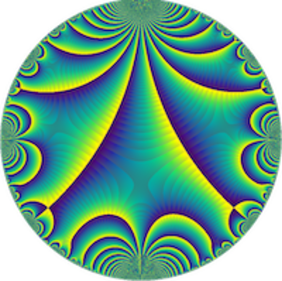# Estimating Sums of Hecke Eigenvalues in a Quadratic Sequence — Alexander Walker (UCL)

Séminaire « Arithmétique »
M2 Kampé de Fériet

Many arithmetic functions which are well-understood on average over sets of positive density remain mysterious when considered over sparser sets. For example, it is not known if there are infinitely many primes of the form n^2 + 1. The behavior of the divisor function on quadratic sequences was first studied by Hooley and refined by Bykovskii. More recently, Blomer has asked a similar question for the Hecke eigenvalues of a holomorphic cusp form. In this talk, we show how to strengthen Blomer's error estimate through the use of shifted convolution sums and the spectral theory of (half-integral weight) automorphic forms.